Next: 6.3.2 Resist/Substrate Interface Up: 6.3 Formulation of the Previous: 6.3 Formulation of the

## 6.3.1 Air/Resist Interface

Above the air/resist interface, i.e., for z > 0, the light consists of incoming Ei, nmpq and reflected waves Er, nmpq (c.f. Figure 6.1). As outlined in Section 4.1.5 in combination with the vector-valued aerial image simulation the incident electric field vector Eipq(x) is given by the Rayleigh expansion of (4.55), whereby the superscript (p, q) refers to the source point considered. Due to the assumption of a periodic simulation domain also the reflected light Erpq(x) can be represented by a Rayleigh expansion. Hence the field distribution above the simulation domain, which is due to one source point, follows from the superposition of two Rayleigh expansions and writes to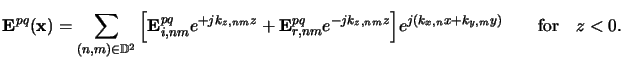(6.28)

Note that we have already used truncated expansions, which is no approximation as long as the set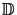2 comprises all incident waves. In any reasonable simulation application this will be the case.

Since the transversal electric field components must be continuous across the interface [11, p. 6] we obtain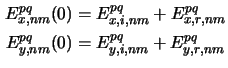for the Fourier coefficients of the lateral electric field components right at the upper boundary at z = 0. With the transverseness property of homogeneous plane waves, i.e., Enm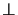Hnmknm, the magnetic wave amplitudes can easily be calculated from the electric ones. The lateral magnetic field components must be continuous, too. Performing the same derivation as in Appendix C that resulted in (C.7), we obtain for the lateral components of the Fourier coefficients of the magnetic field Hpq(x):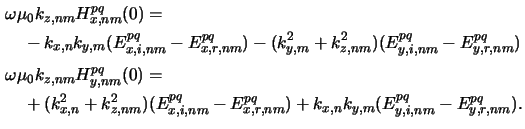The two equations (6.38) and (6.39) establish four relations for each index pair (n, m) of the (2Nx + 1) x (2Ny + 1) Fourier coefficients. This means that we have already derived NODE = 4 x (2Nx + 1) x (2Ny + 1) BCs (cf. (6.29)). However, the lateral coefficients Erx, nm and Ery, nm of the reflected light are unknown in (6.38) and (6.39). Hence we have to eliminate them and end up at NODE/2 conditions for the upper interface z = 0 that write to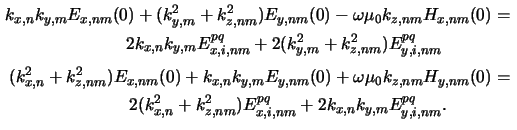To clarify the number of equations that are represented by (6.40): There are (2Nx + 1) x (2Ny + 1) index-pairs (n, m) and two equations, one for each lateral direction x and y. Thus (6.40) represents 2 x (2Nx + 1) x (2Ny + 1) or NODE/2 BCs valid at z = 0.Next: 6.3.2 Resist/Substrate Interface Up: 6.3 Formulation of the Previous: 6.3 Formulation of the
Heinrich Kirchauer, Institute for Microelectronics, TU Vienna
1998-04-17